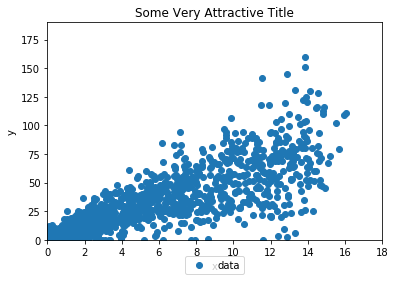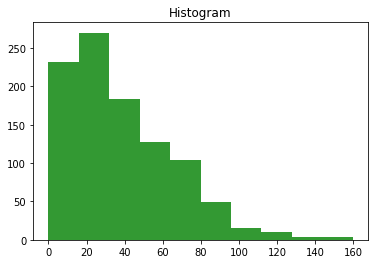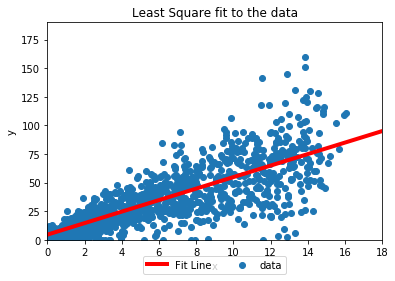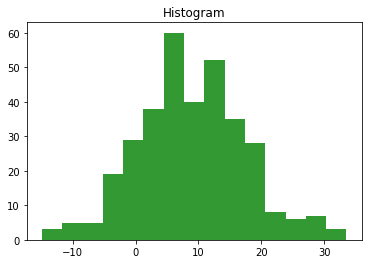# Linear Regression Walkthrough

Back To The Index

01_LinearRegression

## Introduction¶

Regression analysis is a way to develop a mathematical relationship between a set of variables called features and an a resultant variable called target. For example, a business analyst might need to:

• Predict sales for a given level of promotional expenditure.
• Predict electricity demand for a given level of daily peak temperature.
• Predict crime rate based on unemployment and poverty metrics.

We all often rely on intuition to judge how two variables are related. To this point, think of relationship between:

• Gas prices and road accidents.
• S&P Index and apartment rents.
• Crop yields for corn and prices of bacon.

Whatever be your answers to the prompts above, they were all based on extrapolation of historical information (data). Is there a way to formalize and build some sembelance of this intutive reasoning into the algorithms?

Linear Regression was probably historically the first and yet it still is a popular method of establishing relationships between variables. In this tutorial we will learn how to do data exploration and then build a predictive model using Linear Regression Algorithm.

## Linear Regression with Python : A Walkthrough¶

In :
#Load the Libraries
import numpy as np
import scipy as scp
import matplotlib.pyplot as plt
import math

#Setup the path to desktop
desktop = 'C:\\Users\\singa72\\Desktop\\'

In :
### Open the file
datafile = open(desktop+"LinearData.txt",'r')
### First line is just column names
### Read Data line by line
data = [ [float(word) for word in line.split()] for line in datafile.readlines()]
### Check a few lines
print (data[0:3])
### Check total length of loaded data
n = float (len (data))
print ('Total Number of Lines: ',n)
### Close the data file.
datafile.close()

[[6.4063, 45.8259], [1.3291, 14.6734], [11.793, 43.5116]]
Total Number of Lines:  999.0

In :
#### Split the rows in parallel columns dataX and dataY
dataX = [XY for XY in data]
dataY = [XY for XY in data]

In :
xbar = sum(dataX)/n
ybar = sum(dataY)/n
print("Average X: ",xbar," Average Y: ",ybar)

varianceX = sum([pow(x-xbar,2) for x in dataX])/(n-1)
stdevX = math.sqrt(varianceX)
print("x variance:", varianceX, "  x standard deviation:", stdevX)

varianceY = sum([pow(y-ybar,2) for y in dataY])/(n-1)
stdevY = math.sqrt( varianceY )
print("y variance:", varianceY, "  y standard deviation:", stdevY)

covariance = sum( [(x-xbar)*(y-ybar) for x,y in zip(dataX,dataY)] ) / n
correlation = covariance/(stdevX*stdevY)
print("covariance:", covariance, "  correlation:",correlation)

Average X:  6.654674474474473  Average Y:  38.07218038038029
x variance: 17.37421638011934   x standard deviation: 4.16823900227894
y variance: 759.0047184592539   y standard deviation: 27.550040262388983
covariance: 87.24034486952931   correlation: 0.7597006935144369

In :
def splot(dataX,dataY):
fig = plt.figure()
ax = plt.subplot(111)
ax.scatter(dataX, dataY,label='data')
plt.title('Some Very Attractive Title')
plt.xlabel('x')
plt.ylabel('y')
axes = plt.gca()
axes.set_xlim([0,18])
axes.set_ylim([0,190])
ax.legend(loc='upper center', bbox_to_anchor=(0.5, -0.05), shadow=False, ncol=2)
fig.patch.set_facecolor('white') #sets the color of the border
plt.show()
splot(dataX,dataY)In :
def hplot(data, nbins, label=''):
fig = plt.figure()
ax = plt.subplot(111)
ax.hist(data,nbins,color='green',alpha=0.8)
plt.title('Histogram')
plt.xlabel(label)
fig.patch.set_facecolor('white') #sets the color of the border
plt.show()
hplot(dataY,10)### Calculating the regression coefficients.¶

The best estimators for regression coefficients are calculated using least square fit formulae: $$m = \frac{\bar{y} \sum{x^2}-\bar{x}\sum{xy}}{\sum{x^2}-(\sum{x})^2}~~~~~~ b = \frac{\sum{xy}-(\sum{x})(\sum{y})}{n(\sum{x}^2)-(\sum{x})^2}$$

In :
dataX2 = [x*x for x in dataX]
dataY2 = [y*y for y in dataY]
dataXY = [x*y for x,y in zip(dataX,dataY)]

m = ( sum(dataXY)*n - sum(dataX)*sum(dataY)  )/( sum(dataX2)*n - pow(sum(dataX),2)  )
b = ( sum(dataY)*sum(dataX2) - sum(dataX)*sum(dataXY) )/( sum(dataX2)*n - pow(sum(dataX),2)  )

print("m: ",m,"  b: ",b)

m:  5.026284819652386   b:  4.623891089601036


### Visualizing the Model¶

We would like to plot this line on top of the data. To do that, we first have to make some points that follow the line, and then make a scatter plot of dataX vs dataY.

In :
linX = range(20)
linY = [m*x+b for x in linX]

fig = plt.figure()
ax = plt.subplot(111)
ax.scatter(dataX, dataY,label='data')
ax.plot(linX, linY, color='red', label='Fit Line',linewidth=4.0)##New: fit line
plt.title('Least Square fit to the data')
plt.xlabel('x')
plt.ylabel('y')
axes = plt.gca()
axes.set_xlim([0,18])
axes.set_ylim([0,190])
ax.legend(loc='upper center', bbox_to_anchor=(0.5, -0.05), shadow=False, ncol=2)
fig.patch.set_facecolor('white')
plt.show()#### Quantifying the model performance¶

First thing to do with trained model is to check how well fit line explains the data. Contextually, the term residual is used to denote the difference betweenv value y of the dependent variable from model and actual observation. The residuals are used to calculate R^2 metric which is a measure of the goodness of the fit. It tells us what proportion of the variance in Y is explained by the model. For a perfect fit, R^2 would be 1.

In :
SS_residuals = sum( [pow(y - (m*x+b),2) for x,y in zip(dataX,dataY) ]  )
SS_total = sum([pow(y-ybar,2) for y in dataY])
R_squared = 1.0 - (SS_residuals/SS_total)
print('The R^2 Value: ',R_squared)

The R^2 Value:  0.5783023266814258


That’s pretty mediocer, which is to be expected in a system as noisy as this one. We can also select a slice of the plot, or the whole thing, and view the residuals. Again, these are the vertical distances from the fit line.

In :
residuals = [y - m*x+b for x,y in zip(dataX,dataY) if x < 4]
hplot(residuals,15)This gives us a very narrow peak so we can see the spread around our model line.

Back To Index